# Motion in a Straight Line MCQs for NEET

When an object changes its position with respect to its surroundings with time, it is called a motion.

There are three types of motion as follows:

• One dimensional motion
• Two-dimensional motion
• Three-dimensional motion

There are different graphs of motion as follows:

• Displacement-Time Graph
• Velocity-Time Graph
• Acceleration-Time Graph

Following are the equations of uniformly accelerated motion:

• v= u+at
• s=ut+at2
• v2=u2+2as

1. What is the acceleration if the body starts from rest and travels a distance of ‘s’ m in 2 seconds?

1. $$(\frac{2}{3}s)\,m/s^2$$
2. $$(\frac{3}{2}s)\,m/s^2$$
3. $$(\frac{1}{3}s)\,m/s^2$$
4. $$(\frac{s}{2})\,m/s^2$$

Answer: (d)$$(\frac{s}{2})\,m/s^2$$

2. What is the ratio of the average acceleration during the intervals OA and AB in the velocity-time graph as shown below?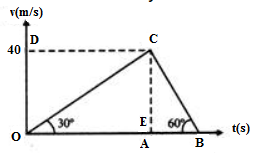1. ½
2. 1
3. 3

3. A body covers 16, 18, 20, 22 meters in 5th, 6th, 7th and 8th seconds respectively. Which of the following statements is true about the body?

1. The body moves with a uniform velocity from rest
2. The body from rest moves with uniform acceleration
3. The body moves with an initial velocity and moves with uniform acceleration
4. The body moves with an initial velocity and then moves with uniform velocity

Answer: (c) The body moves with an initial velocity and moves with uniform acceleration

4. What can be said about the displacement of the body if it covers a distance of zero?

1. It is zero
2. It cannot be zero
3. It may or may not be zero
4. It is negative

5. An athlete finishes a round of circular track of radius R in 40 sec. What is his displacement at the end of 2 min 20 sec?

1. 2R
2. 2πR
3. 7πR
4. Zero

6. Which of the following is not possible for a body in uniform motion?

1.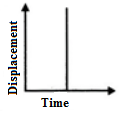2.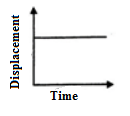3. (c) Both (a) & (b)
4. (d) None of the above

Answer: (c) Both (a) & (b)

7. If a car travels ⅖ th of the total distance with a speed v1 and ⅗ th of the distance with a speed v2. The average speed is given by

1. $$\frac{2v_{1}v_2}{v_{1}+v_2}$$
2. $$\frac{1}{2}\sqrt{v_1v_2}$$
3. $$\frac{5v_{1}v_2}{3v_{1}+2v_2}$$
4. $$\frac{v_{1}+v_2}{2}$$

Answer:(c) $$\frac{5v_{1}v_2}{3v_{1}+2v_2}$$

8. A man leaves home for a cycle ride and comes back home after an half-an-hour ride covering a distance of one km. What is the average velocity of the ride?

1. 10 kms-1
2. ½ kms-1
3. 2 kms-1
4. Zero

9. A bird flies with a speed of 10 km/hr and a car moves with uniform speed 8 km/hr. Both start from B to A at the same instant (BA = 40 km). The bird after reaching A flies back immediately to meet the approaching car. Once it reaches the car, it flies back to A. The bird repeats this until both the car and the bird reach A simultaneously. What is the total distance flown by the bird?

1. 80 km
2. 50 km
3. 40 km
4. 30 km

10. Elephants A, B and C move with a constant speed in the same direction along a straight line as shown in the figure. The speed of the elephant A is 5 m/s and the speed of the elephant C is 10 m/s. Initially, the separation between A and B is d and between B and C is also d. When B catches C, the separation between A and C becomes 3d. What will the speed of B be?

1. 5 m/s
2. 7.5 m/s
3. 15 m/s
4. 20 m/s

11. Which of the following remains constant if a body travels with constant acceleration?

1. Time
2. Velocity
3. Displacement
4. None of the above

Answer: (d) None of the above

12. Given here is a velocity-time graph of the motion of a body. What is the distance travelled by the body during the motion?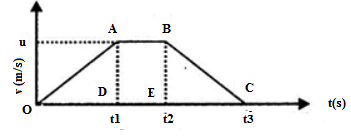1. $$\frac{1}{2}(OA+AB)\times BC$$
2. $$\frac{1}{2}(OA+BC)\times OC$$
3. $$\frac{1}{2}(OC+AB)\times AD$$
4. $$\frac{1}{2}(AD+BE)\times OC$$

Answer: (c) $$\frac{1}{2}(OC+AB)\times AD$$

13. Which of the following graphs gives the equation $$x=v_{0}t+\frac{1}{2}at^2$$

1.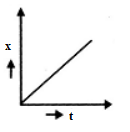2.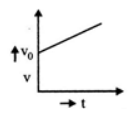3.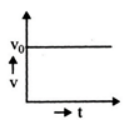4. None of the above14. What does the displacement of the particle shown in the graph indicate?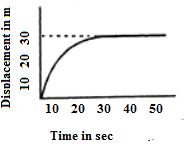1. It indicates a constant velocity
2. It indicates a constant acceleration
3. It indicates that the particle starts with a constant velocity and is accelerated
4. It indicates that the motion is retarded and the particle stops

Answer: (d) It indicates that the motion is retarded and the particle stops

Stay tuned to BYJU’S and Fall in Love with Learning!

Check out these NEET Physics MCQs: